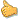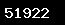## STRUCTURED QUERY LANGUAGE(SQL)

Lesson Purpose:This lesson is designed to develop students’ understanding of relational databases

and structured query language.
Lesson Purpose:
This lesson is designed to develop students’ understanding of relational databases
and structured query language.
Lesson Objectives:Identify the main components of a relational database.Identify and demonstrate the proper use of basic SQL statements.Identify and demonstrate the proper use of wildcards and SQL operators.Identify and demonstrate the proper use of the SQL Clauses.Lesson Activity #1 (Introduction):Have a volunteer demonstrate problem that requires the use of SQL to correct. Introduce the
objectives of the course and the expectations of each student.
Lesson Activity 1:Have students break into groups of 3. Instruct each group to utilize the web sites listed below to
develop a 15-minute presentation related to the main components of a relational database.
Have each group present their finding to the other groups.
Lesson Activity 2:Have each student complete Tasks 1-9 of the SQL Interpreter and Tutorial from the website listed
below. Discuss what The students learned about the basic SQL statements. Explain/Demonstrate
how the SQL statements learned on the tutorial are applied to the your organizations database.
http://www.sqlcourse.com/

Lesson Activity 3:Have each student complete Task 10 of the SQL Interpreter and Tutorial. Discuss what the students
how the SQL statements learned on the tutorial are applied to your organizations database.
Lesson Activity 4 :Review the objectives covered. Have each student complete an exam testing their knowledge
of the basic SQLstatements, clauses, etc. Have each student subscribe to the SQL discussion group listed below
, which they can utilize on the job when they have SQL related questions and to further their
understanding of SQL. This activity also containselements of the INFORM strategy.Identify the main components of a relational database.Identify and demonstrate the proper use of basic SQL statements.Identify and demonstrate the proper use of wildcards and SQL operators.Identify and demonstrate the proper use of the SQL Clauses.
Lesson Activity 5 (Introduction):Have a volunteer demonstrate problem that requires the use of SQL to correct. Introduce the
objectives of the course and the expectations of each student.
Lesson Activity 6:Have students break into groups of 3. Instruct each group to utilize the web sites listed below
to develop a 15-minute presentation related to the main components of a relational database.
Have each group present their finding to the other groups.

Lesson Activity 7:Have each student complete Task 10 of the SQL Interpreter and Tutorial. Discuss what the studentsReview the objectives covered. Have each student complete an exam testing their knowledge of the